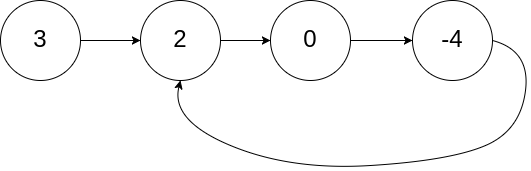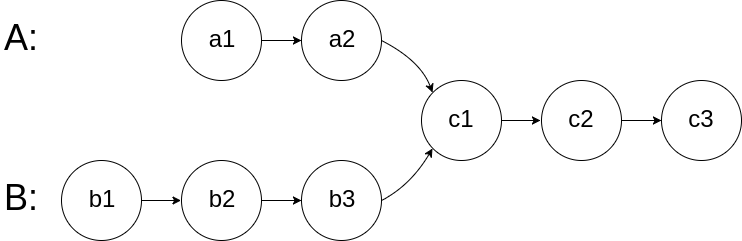# Dynamic Programming - 279. Perfect Squares

279. Perfect Squares Given a positive integer n, find the least number of perfect square numbers (for example, 1, 4, 9, 16, ...) which sum to n. Example 1: Input: n = 12 Output: 3 Explanation: 12 = 4 + 4 + 4. Example 2: Input: n = 13 Output: 2 Explanation: 13 = 4 + 9. 思路： 题目意思是求能用最少的完全平方表示一个数，有一个四平方和定理，可以在O(n)内解决问题，但是这里选择用动态规划来做，子问题就是一个数是由另一个数加上一个平方数，也就是比如A = B + x^2, 而B由是子问题求解，这样的话，能表示A的最少组合就是B最少组合加一，所以动态转移方程就是：dp[i] = min{dp[ i - j*j] + 1}, (j*j <= i),初始条件是dp = 0....# Dynamic Programming - 120. Triangle

120. Triangle Given a triangle, find the minimum path sum from top to bottom. Each step you may move to adjacent numbers on the row below. For example, given the following triangle [ , [3,4], [6,5,7], [4,1,8,3] ] The minimum path sum from top to bottom is 11 (i.e., 2 + 3 + 5 + 1 = 11). Note: Bonus point if you are able to do this using only O(n) extra space, where n is the total number of rows in the triangle. 思路： 题目意思是给一....# Dynamic Programming - 63. Unique Paths II

63. Unique Paths II A robot is located at the top-left corner of a m x n grid (marked ‘Start’ in the diagram below). The robot can only move either down or right at any point in time. The robot is trying to reach the bottom-right corner of the grid (marked ‘Finish’ in the diagram below). Now consider if some obstacles are added to the grids. How many unique paths would there be? An obstacle and empty space is marked as 1 and 0 respectively in the gri....# Dynamic Programming - 62. Unique Paths

62. Unique Paths A robot is located at the top-left corner of a m x n grid (marked ‘Start’ in the diagram below). The robot can only move either down or right at any point in time. The robot is trying to reach the bottom-right corner of the grid (marked ‘Finish’ in the diagram below). How many possible unique paths are there? Above is a 7 x 3 grid. How many possible unique paths are there? Note: m and n will be at most 100. Example 1: Input: m = 3, ....# Dynamic Programming - 70. Climbing Stairs

70. Climbing Stairs You are climbing a stair case. It takes n steps to reach to the top. Each time you can either climb 1 or 2 steps. In how many distinct ways can you climb to the top? Note: Given n will be a positive integer. Example 1: Input: 2 Output: 2 Explanation: There are two ways to climb to the top. 1. 1 step + 1 step 2. 2 steps Example 2: Input: 3 Output: 3 Explanation: There are three ways to climb to the top. 1. 1 step + 1 step + 1 step 2. 1 step + 2....# LinkedList - 23. Merge k Sorted Lists

Merge k sorted linked lists and return it as one sorted list. Analyze and describe its complexity.# LinkedList - 86. Partition List

86. Partition List Given a linked list and a value x, partition it such that all nodes less than x come before nodes greater than or equal to x. You should preserve the original relative order of the nodes in each of the two partitions. Example: Input: head = 1->4->3->2->5->2, x = 3 Output: 1->2->2->4->3->5 思路： 题目意思是给一个链表和一个数字，将链表前半部分变成小于这个数字后半部大于等于这个数字。考的是链表基本操作。使用两个指针，记录下比输入小的链表和大于等于该数字的链表，再把两个链表接起来。 代码： java： /** * Definition for sing....# LinkedList - 148. Sort List

148. Sort List Sort a linked list in O(n log n) time using constant space complexity. Example 1: Input: 4->2->1->3 Output: 1->2->3->4 Example 2: Input: -1->5->3->4->0 Output: -1->0->3->4->5 思路： 题目意思很明确，给一个链表排序，排序的方式有很多，这里写一下归并排序。 代码： java： /** * Definition for singly-linked list. * public class ListNode { * int val; * ListNode next; * ListNode(int x) { val = x; } * } */ class Solution { public ListNode sortList(ListNode head) { ....# LinkedList - 61. Rotate List

61. Rotate List Given a linked list, rotate the list to the right by k places, where k is non-negative. Example 1: Input: 1->2->3->4->5->NULL, k = 2 Output: 4->5->1->2->3->NULL Explanation: rotate 1 steps to the right: 5->1->2->3->4->NULL rotate 2 steps to the right: 4->5->1->2->3->NULL Example 2: Input: 0->1->2->NULL, k = 4 Output: 2->0->1->NULL Explanation: rotate 1 steps to the right....Given a linked list, return the node where the cycle begins.# LinkedList - 143. Reorder List

143. Reorder List Given a singly linked list L: L_0→_L_1→…→_L__n-1→_L_n, reorder it to: L_0→_L__n_→_L_1→_L__n-1→_L_2→_L__n_-2→… You may not modify the values in the list’s nodes, only nodes itself may be changed. Example 1: Given 1->2->3->4, reorder it to 1->4->2->3. Example 2: Given 1->2->3->4->5, reorder it to 1->5->2->4->3. 思路： 题目意思是给一个链表，然后重新按照一个前一个后的顺序重新排序。题目主要是考链表的操作，比如找到链表的终点，然后翻转链表，然后交换链表的节点，考的就是链表操作基本功。注意找中点的时候，找到的....160. Intersection of Two Linked Lists Write a program to find the node at which the intersection of two singly linked lists begins. For example, the following two linked lists: begin to intersect at node c1. Example 1: ``` Input: intersectVal = 8, listA = [4,1,8,4,5], listB = [5,0,1,8,4,5], skipA = 2, skipB = 3 Output: Reference of the node with value = 8 Input Explanation: The intersected node’s value is 8 (note that this must not be 0 if the two lists intersect). From the head of A, it r....# LinkedList - 21. Merge Two Sorted Lists

Merge two sorted linked lists and return it as a new list. The new list should be made by splicing together the nodes of the first two lists.2. Add Two Numbers You are given two non-empty linked lists representing two non-negative integers. The digits are stored in reverse order and each of their nodes contain a single digit. Add the two numbers and return it as a linked list. You may assume the two numbers do not contain any leading zero, except the number 0 itself. Example: Input: (2 -> 4 -> 3) + (5 -> 6 -> 4) Output: 7 -> 0 -> 8 Explanation: 342 + 465 = 807. 思路： 题目意思是给两个链表，把这两个链表，一个链表代....# LinkedList - 82. Remove Duplicates from Sorted List II

82. Remove Duplicates from Sorted List II Given a sorted linked list, delete all nodes that have duplicate numbers, leaving only distinct numbers from the original list. Example 1: Input: 1->2->3->3->4->4->5 Output: 1->2->5 Example 2: Input: 1->1->1->2->3 Output: 2->3 思路： 题目意思是移除所有重复过的节点，做法就是得要找到当前节点和下一个节点不相同的节点，然后把链表接上，跳过重复的节点。 代码： java： /** * Definition for singly-linked list. * public class ListNode { * int val; * ListNode next; * Li....203. Remove Linked List Elements Remove all elements from a linked list of integers that have value val. Example: Input: 1->2->6->3->4->5->6, val = 6 Output: 1->2->3->4->5 思路： 移除制定的节点，需要注意的是，如果移除的是第一个节点，也需要移除。 代码： java： /** * Definition for singly-linked list. * public class ListNode { * int val; * ListNode next; * ListNode(int x) { val = x; } * } */ class Solution { public ListNode removeElements(ListNode head, int val) { if (head == null) return head....# LinkedList - 83. Remove Duplicates from Sorted List

83. Remove Duplicates from Sorted List Given a sorted linked list, delete all duplicates such that each element appear only once. Example 1: Input: 1->1->2 Output: 1->2 Example 2: Input: 1->1->2->3->3 Output: 1->2->3 思路： 这一题和26题移除数组中重复的元素一样都是去重，只不过这里的数据是链表，思路就是判断当前节点数值和下一个的节点值是否相同，如果相同就跳过下一个节点。不同就移动指针到下一个节点继续判断。 代码： java： /** * Definition for singly-linked list. * public class ListNode { * int val; * ListNode next; * ListNode(int x) { val = x; } * } */ ....# LinkedList - 19. Remove Nth Node From End of List

19. Remove Nth Node From End of List Given a linked list, remove the n-th node from the end of list and return its head. Example: Given linked list: **1->2->3->4->5**, and **_n_ = 2**. After removing the second node from the end, the linked list becomes **1->2->3->5**. Note: Given n will always be valid. Follow up: Could you do this in one pass? 思路： 删除链表倒数第n个节点，就是要走到倒数第n+1个节点进行操作，如果一次遍历解决问题，就得使用两个指针，两个指针开始都在同一个位置，然后fast指针先走n+1步，然后slow指针开始和fast指针一起走，这样fa....